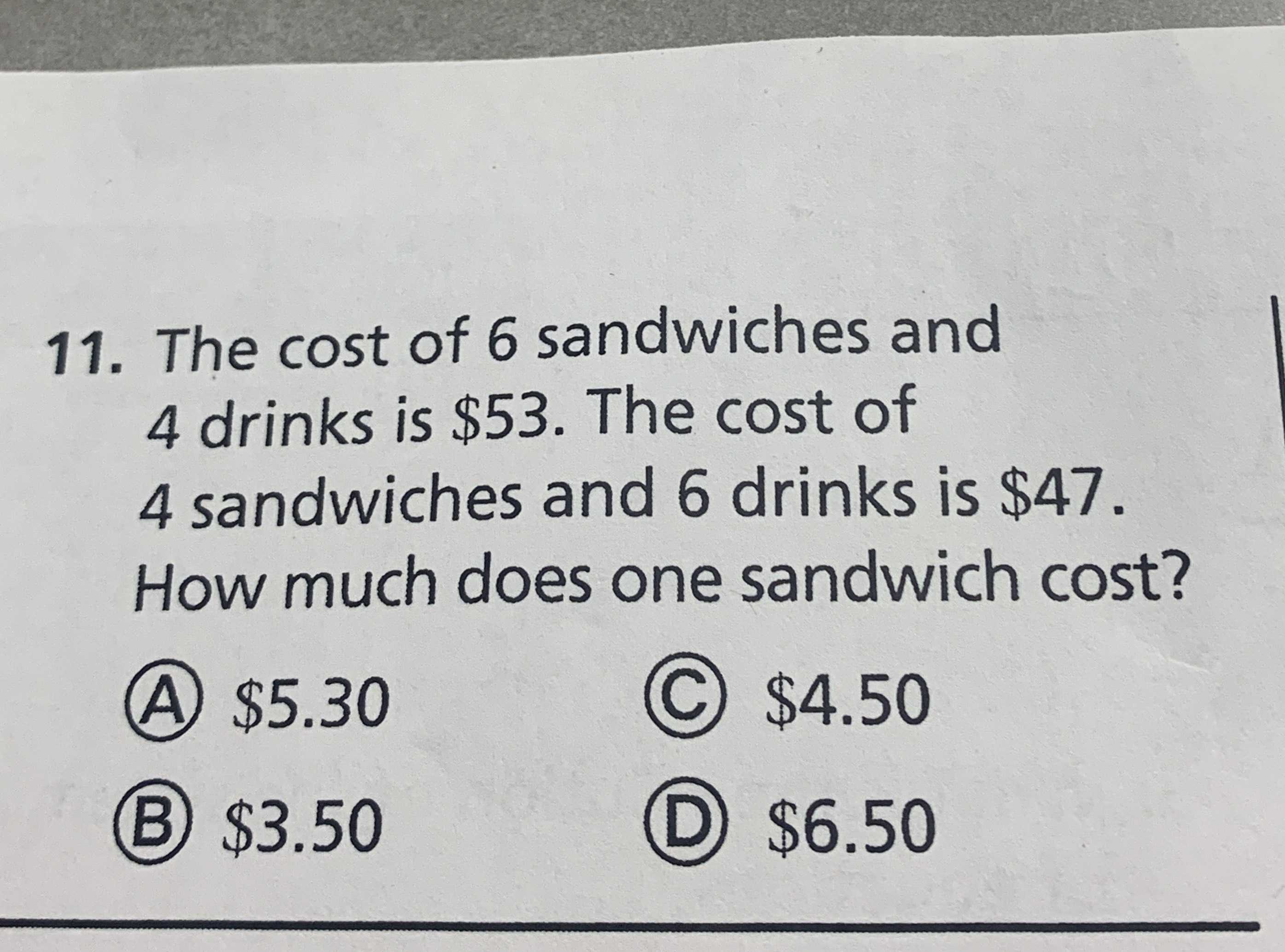### ¿Todavía tienes preguntas de matemáticas?

Pregunte a nuestros tutores expertos
Algebra
Pregunta11. The cost of $$6$$ sandwiches and $$4$$ drinks is $$\ 53$$ . The cost of $$4$$ sandwiches and $$6$$ drinks is $$\ 47 .$$ How much does one sandwich cost?

(A) $$\ 5.30$$ (C) $$\ 4.50$$

(B) $$\ 3.50$$ (D) $$\ 6.50$$